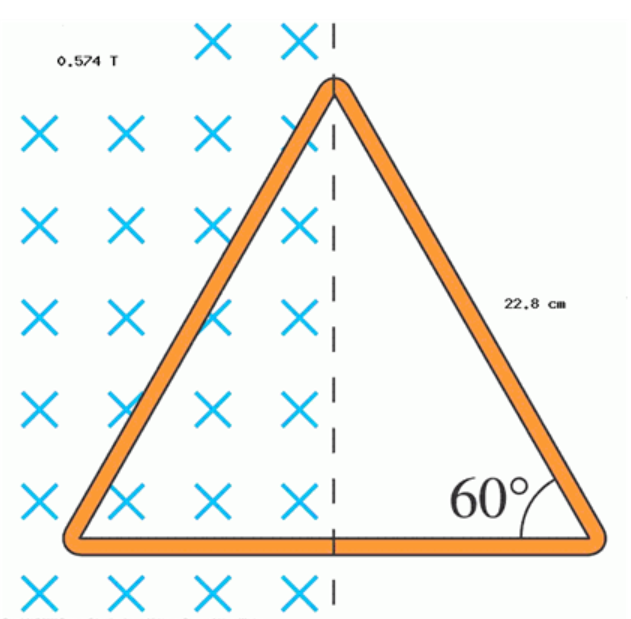# Problem: The metal equilateral triangle in the diagram above, 22.8 cm on a side, is halfway into a 0.574 T magnetic field. What is the magnetic flux (in Wb) through the triangle?

###### FREE Expert Solution

Magnetic flux:

$\overline{){{\mathbf{\varphi }}}_{{\mathbf{B}}}{\mathbf{=}}{\mathbf{B}}{\mathbf{A}}{\mathbf{c}}{\mathbf{o}}{\mathbf{s}}{\mathbf{\theta }}}$

A is the area of half the triangle, which is:

A = (1/2)[(1/2)(22.8 × 10-2)][sin (60°) (22.8 × 10-2)] = 0.1125 m2

98% (361 ratings)###### Problem DetailsThe metal equilateral triangle in the diagram above, 22.8 cm on a side, is halfway into a 0.574 T magnetic field. What is the magnetic flux (in Wb) through the triangle?